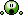## Deviation Actions

10K Views
submission for Evokeone's pack XXI "Spectrum"

Image details
Image size
566x800px 228.05 KB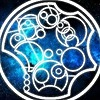Something to try, as far as mathematical operations go you could almost plot a x axis for them but here is their names and their likewise reperesntative numbers. Planckation (the 10^-35 operation) Yoctation (the 10^-24 operation), Zeptation (the 10^-21 operation), Autation (the 10^-18 operation), Femptation (the 10^-15 operation), Pication (the -1,000,000,000,000 operation), Nanation (the -1,000,000,000 operation), Micration (the -1,000,000 operation), Millation (the -1,000 operation), Centation (the -100 operation), Negative Decation (the -10 operation), Noventation (the -9 operation), Negative Octation (the -8 operation), Septation (the -7 operation), Sexation (the -6 operation), Quintation (the -5 operation), Quadation or Negative﻿ Tetration (the -4 operation), Negative Exponentiation (the -3 operation), Division (the -2 operation), Subtraction (the -1 operation), Zeration (the 0 operation), Addition (the 1st operation), Multiplication (the 2d operation), Exponentiation (the 3d operation), Tetration (the 4th operation), Pentation (the 5th operation), Hexation (the 6th operation), Heptation (the 7th operation), Octation (the 8th operation), Nonation (the 9th operation), Decation (the 10th operation), Undecation (the 11th operation) Hectation (the 100th operation), Kilation (the 1,000th operation), Megation (the 1,000,000th operation), Gigation (the 1,000,000,000th operation), Terration (the 1,000,000,000,000th operation), Petation (the 10^15 operation), Exation (the 10^18 operation), Zettation (the 10^21 operation), Yattation (the 10^24 operation), Xonation (Xona meaning Octillion the 10^27 operation), Wekation (Weka meaning Nonillion the 10^30 operation), Yundation (Yunda meaning Decillion the 10^33 operation). You could imagine further operations like Ninetation (The Ninetillionth operation, Ninetillion being 10^3x10^3x10^270+3), Palation (the Palillionth operation, Palillion being 10^(3x10^(3x10^15,000) +3)), Multation (The Multillionth level of notation, Multillion being 10^(3x10^(3x10^3tredecillion) +3)), Giggation (The Giggolth operation, Giggol being 10 Tetrated to 100), Megastation (The Megastillionth operation, Megaston being roughly 10 Pentated to 11), Triseptation (The Triseptillionth operation, Trisept being 7 Heptated to 7), Goggation (The Goggillionth operation, Goggol being {10,100,8}), Biggation (The Biggolth operation, Biggol being {10,10,100,2}), Baggation (The Baggolth operation, Baggol being {10,10,100,3}), Troogation (The Troogolth operation, Troogol being {10,10,10,100}), Hexatration (The Hexatrillionth operation, Hexatri being {3,3,3,3,3,3}), Quintigation (The Quintigillionth operation, Quintigol being {10,10,10,10,10,100,5}), Gaitration (The Gaitrillionth operation, Gaitrol being {10,100,7 (1) 3}) or even Mussation (The Mussationth operation, Mussol being {10,10 (1) 10,100,5}). What this means is as you get to higher enough notations you need to eventually exponentiation or even tetrate the number of notations instead of notating an actual number but once you Decate, Megate even Yundate the level of operations you start a third layer of Exponentiation, Tetration even Gigation. Eventually these layers become so numerous that a whole series of operations needs to be invented up to even Hexatration to notate the number of operation levels not of number or of notation layers but a third dimention of operations. This goes even for negative number but mostly for positive ones. Think about that.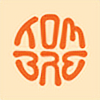thanks for info, some of the names sound really interesting, I would never guess they mean something in math, like Mussation or Baggation.Well those funny names are not official operations but they can be made and those numbers do exist so put that with the corresponding operation and that is what would happen thanks.if you dont mind me asking, could you do a quick little look up and tell me how many layers this took??? i think i may use this as a reference for techniques on a future project...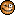well, the illu is kinda complicated shape because of all those lines and so on, the ps are all the masks and blends, i usually work with many far more layers than i really need, because i keep some backup if wanted to return to some previous step... almost nothing drawn in ps, so its like 40-50 layersThank you very much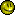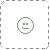very nice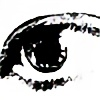featured here: [link]this is ill, I want a poster of it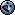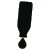One of the best in the release man, I like how you've divided the colours.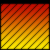great!!!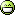krása kamo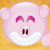wow aweosem design...as usual!